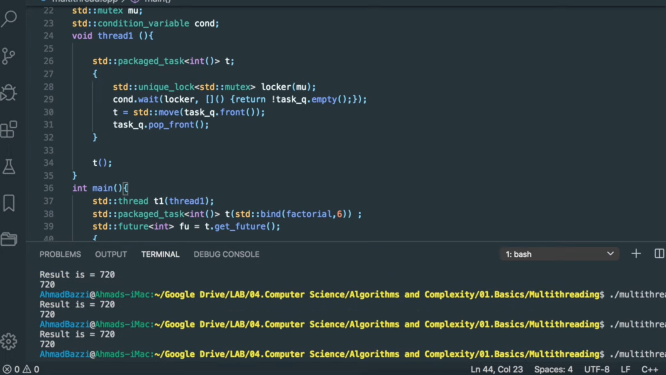• Last Updated : 31 Jan, 2022

The  std::packaged_task class wraps any Callable objects (function, lambda expression, bind expression, or another function object) so that they can be invoked asynchronously. A packaged_task won’t start on its own, you have to invoke it, As its return value is stored in a shared state that can be called/accessed by  std::future objects.

The main advantage of a packaged task is that it can link a callable object to a future and that is very important in a flooding environment. For example, if we have an existing function that fetches the data from Database (DB) and returns it. Now there is a need to execute this function in a separate thread. This can be done using:

Otherwise, we’ll have to use:

std::promise<>

and have to change code but with the help of  std::packaged_task<> its simple and we don’t need to do that.

Member Functions

Some of the member functions in packaged_task are:

• Operator=- it moves packaged tasks and it’s a public member function.
• Swap- It just swaps to the packaged task or you can say exchange two packaged tasks with each other.
• get_future- It returns a std::future associated with the promised result.
• reset- This public member function just resets the task.
• (constructor)- As the name suggests this public member function constructs the packaged task.
• (destructor)- Similarly, (destructor) destructs the task object.

Non-Member Functions

One of the non-member functions is:

• swap(packaged_task)- It specializes the std::swap algorithm.

Below is the C++ program to implement the above functions-

## C++

 `// C++ program to implement``// the functions``#include`` ` `using` `namespace` `std;`` ` `// Factorial function``int` `factorial(``int` `N)``{``    ``int` `res = 1;``    ``for` `(``int` `i = N; i > 1; i--) ``    ``{``        ``res *= i;``    ``}``   ` `    ``cout << ``"Result is = "` `<< ``             ``res << ``"/n"``;``    ``return` `res;``}`` ` `// packaged task``std::deque > task_q;    ``std::mutex mu;``std::condition_variable cond;`` ` `void` `thread1() ``{``  ``// packaged task``  ``std::packaged_task<``int``()> t;                       ``  ``{``    ``std::unique_lock locker(mu);``    ``cond.wait(locker, []() ``              ``{ ``                ``return` `!task_q.empty();``              ``}); ``    ``t = std::move(task_q.front());``    ``task_q.pop_front();``  ``}  ``  ``t();``}`` ` `// Driver Code``int` `main()``{``      ``std::``thread` `t1(thread1);``     ` `    ``// Create a packaged_task<> that ``    ``// encapsulated the callback i.e. a function``    ``std::packaged_task<``int``()> t(bind(factorial,6));    ``   ` `    ``// Fetch the associated future<> ``    ``// from packaged_task<>``      ``std::future<``int``> fu = t.get_future();              ``  ``{``    ``std::lock_guard locker(mu);``    ``task_q.push_back(std::move(t));``  ``}``   ` `      ``cond.notify_one();``   ` `    ``// Fetch the result of packaged_task<>``    ``cout<

Output:My Personal Notes arrow_drop_up